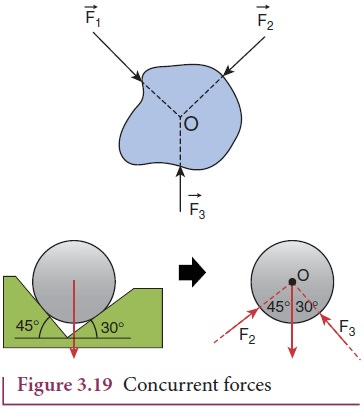Home | | Physics 11th std | Application of Newton’s Laws: Concurrent Forces and Lami’s Theorem

# Application of Newton’s Laws: Concurrent Forces and Lami’s Theorem

A collection of forces is said to be concurrent, if the lines of forces act at a common point.

Concurrent Forces and Lami’s Theorem

A collection of forces is said to be concurrent, if the lines of forces act at a common point. Figure 3.19 illustrates concurrent forces.

Concurrent forces need not be in the same plane. If they are in the same plane, they are concurrent as well as coplanar forces.Study Material, Lecturing Notes, Assignment, Reference, Wiki description explanation, brief detail
11th Physics : UNIT 3 : Laws of Motion : Application of Newton’s Laws: Concurrent Forces and Lami’s Theorem |